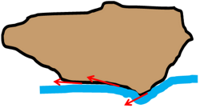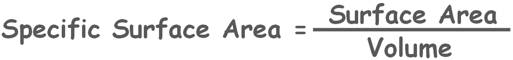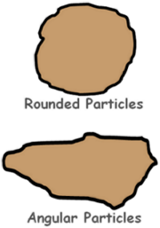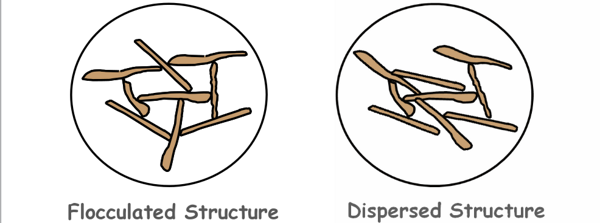Factors Affecting Permeability Of SoilA soil mass is composed of small solid particles which we call the soil grains. These soil grains when depositing in a soil mass arranges themselves in a way that some amount of empty space is left between them. We call these spaces voids.

And the property of the soil which permits the water or any liquid to flow through it through its voids is called permeability. Permeability is the ease with which water can flow through the soils.

Coefficient of permeability of a soil depends on both the properties of the soil and properties of the water which is flowing through it.

Let us discuss some of those. But before that let us consider this equation which is the general expression for the coefficient of permeability.It has been obtained by comparing Poiseuille equation with Darcy’s equation:

Particle size:

So let’s begin with particle size. When a soil mass contains coarse grained particles it contains large volume of voids and those voids are interconnected. So, high amount of water may flow through these interconnected voids easily. Hence such soils have higher value of permeability.

While soil mass with fine grains have poorly connected void structure consequently we observe lower value of permeability.We can also notice in the general equation that permeability of the soil is directly proportional to the square of particle diameter.

If the soil particle size is large its permeability will be high and if particle size is small the permeability will be low.

Specific surface area of soil particles:The surface of the soil particles offers a resistance to the flow of water. The more surface is in contact with the flowing water greater is the resistance posed to the water. Hence lower will be the permeability.

Specific surface area is the total surface area of the particle divided by its volume.If volume of a particle is kept constant but its surface area is increased then specific surface area of that particle will also increase.

Coarse grained particles have relatively less specific surface area so they pose less resistance to flow of water consequently offer relatively high coefficient of permeability, while fine soil particles have larger specific surface area so resistance will be more hence permeability will be less.

So the permeability is inversely proportional to the specific surface.

Shape of soil particles:Specific surface area of a particle also depends on its shape. Hence permeability also depends on the shape of the soil particles.

Rounded soil particles have relatively less specific surface area when compared to angular particles.

So when water flows through soil mass consists of rounded particles it will face less resistance and permeability will be relatively higher than if water flows through soil mass of angular particles.

Soil Structure:

Permeability also depends on how these particles arrange themselves in a soil mass that is in the structure of the soil mass. Water flows through voids and the connectivity of these voids depends upon the structural arrangement of the soil particles.

In case of fine grained soils if the soil particles are arranged in flocculated structure then its permeability will be more when compared to that if the particles are arrange in the dispersed structure as we know flocculated structure has more voids than in the dispersed structure.If a soil mass has stratified structure then its permeability varies according to the direction of flow. It has been observed that when the flow of water is parallel to the bedding planes the permeability of the soil mass is higher than when water flows perpendicular to the bedding planes.

Including these factors permeability of soil deposit also depends upon the structural defects like cracks or fissures in the soil mass.

Void Ratio:

Void ratio of a soil mass is the volume of voids present in it divided by the volume of solids.If volume of voids in the soil increases the flow path becomes wider and voids interconnectivity increases. Hence permeability of soil increases.

So in general we can say permeability of a soil mass increases with the increase in its void ratio and decreases when voids ratio decrease.

But it is not true for all types of soils. Clay soils have higher void ratio because of their flocculated structure but still their permeability is very low because the flow path through voids in case of clays is extremely small and poorly connected.Fine grained soil particles carry charges on their surface and because of that dipolar water molecules are strongly attached to their surface. This attached water is called adsorbed water. As this water is bounded by electrical forces it is not free to move under gravity.

This adsorbed water layer causes an obstruction to the flow of water in the pores by blocking the voids or reducing their effective size and hence reduces the permeability of soils.

Degree of Saturation:The saturation of the soil mass also affects its permeability. If the soil is partially saturated then it may have some voids which contain entrapped air. These entrapped air pockets may block the flow path which may reduce the permeability of the soil. While if the soil is fully saturated there will not be any such blockage.

Hence we can say the permeability of a partially saturated soil is smaller than that of fully saturated soil.

Water properties:

Permeability of the soil not only depends on the soil properties but also depends upon the properties of its permeant that is water.

Viscosity:

Let us consider the viscosity of the water. If we look at the general permeability equation we notice that permeability is inversely proportional to the viscosity of the fluid.The more viscous the liquid is more resistance it will pose to flow and slower it will move into the voids. Consequently lower will be the permeability.

On the other hand if the liquid flowing through the soil is less viscous, less resistance it will offer to flow hence higher will be the permeability.

Temperature:

With it let’s consider the temperature of the liquid. Permeability is dependent on the viscosity and we know viscosity is inversely dependent on the temperature. Hence, Permeability is directly related to temperature.We know as the temperature of the liquid increases its viscosity decreases, consequently it permeability increases. Similarly when temperature of the liquid flowing through soil decreases, its viscosity increases hence its permeability decreases.

There is a relationship between temperature of the permeant and the permeability of the soil which can be given as this:Here k27 and KT are permeability of soil at temperature 27 degree and test temperature T degree centigrade respectively. And μ27 and μT are viscosity of liquid at temperature and 27 degree and test temperature T degree centigrade respectively.

Impurities and Organic Matter in water:

The presence of impurities or any organic matter in the water or in the soil tends to block the flow path by blocking the voids that result in decreased permeability of the soil.

Tags : permeability

Published on :2020-05-04